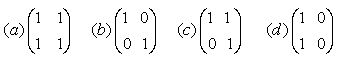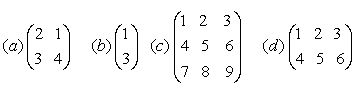Email us to get an instant 20% discount on highly effective K-12 Math & English kwizNET Programs!

#### Online Quiz (WorksheetABCD)

Questions Per Quiz = 2 4 6 8 10

### High School Mathematics - 26.13 Matrix Revision - II

 1. Identity Matrix:- A square matrix in which each element along the principal diagonal is '1' and all the other elements are zero is called a identity matrix or unit matrix. 2. Square matrix:- In a matrix if the number of rows is equal to the number of columns it is called a square matrix. 3. Scalar Matrix:- A square matrix in which all the element in the principal diagonal are equal and the rest of the elements are zeros is called a scalar matrix. 4. Transpose of a Matrix:- The matrix obtained by interchanging the rows and columns of a given matrix is called the transpose of the given matrix. 5. Skew Symmetric Matrix:- If the transpose of a matrix is equal to the additive inverse of a given matrix, then the given matrix is called "Skew symmetric matrix" 6. If A and B are two matrices such that the number of columns in A is equal to the number of rows in B, then only matrix multiplication is possible in A and B. 7. Two matrices are equal if and only if they are of the same order and their corresponding elements are equal. Directions: Answer the following questions. Also write five examples of each matrix.
 Q 1: One of the following is an identity matrix.bcda Q 2: A matrix in which the number of rows is not equal tot he number of columns is called a _____ matrix.RectangularSquare Q 3: If a matrix is equal to its transpose it is called a ______ matrix.Skew symmetricSymmetric Q 4: A matrix in which each element is zero is called a ______ matrix.identityzero Q 5: One of the following is a 3 ´ 3 matrix.bdca Q 6: An identity matrix is a square matrix, in which the elements of the principal diagonal are each '1' and all other elements are zero.FalseTrue Question 7: This question is available to subscribers only! Question 8: This question is available to subscribers only!

#### Subscription to kwizNET Learning System offers the following benefits:

• Unrestricted access to grade appropriate lessons, quizzes, & printable worksheets
• Instant scoring of online quizzes
• Progress tracking and award certificates to keep your student motivated
• Unlimited practice with auto-generated 'WIZ MATH' quizzes